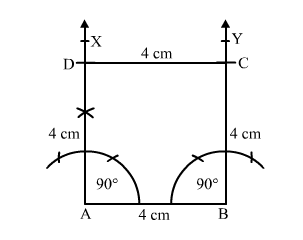# Construct a square of side 4 cm.

Question:

Construct a square of side 4 cm.

Solution:Steps of construction:
1. Draw a line segment AB = 4 cm.

2. Construct $\angle B A X=90^{\circ}$ and $\angle A B Y=90^{\circ}$.

3. Set off AD = 4 cm and BC = 4 cm.
4. Join DC.

Thus, $\square A B C D$ is the required square.